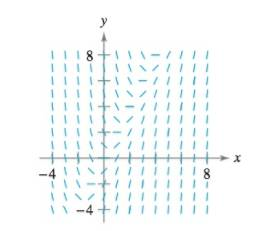Chapter 6, Problem 9RE

Chapter
Section
Textbook Problem

Slope Field In Exercises 9 and 10, a differential equation and its slope field are given. Complete the table by determining the slopes (if possible) in the slope field at the given points. x -4 -2 0 2 4 8 y 2 0 4 4 6 8 d y / d x d y d x = 2 x − yTo determine
The values in the table by determining the slope in the slope field for dydx=2xy.

Explanation

Given:

The function dydx=2xy

The graph:

Explanation:

Let f(x,y)=2xy.

The value of f at the point (4,2) is:

f((4),2)=2(4)2=82=10

So, the value of dydx at the point (4,2) is 10.

The value of f at the point (2,0) is:

f((2),0)=2(2)(0)=4

So, the value of dydx at the point (2,0) is 4.

The value of f at the point (0,4) is:

f(0,4)=2(0)(4)=4

So, the value of dydx at the point (0,4) is 4

Still sussing out bartleby?

Check out a sample textbook solution.

See a sample solution

The Solution to Your Study Problems

Bartleby provides explanations to thousands of textbook problems written by our experts, many with advanced degrees!

Get Started

Find more solutions based on key concepts